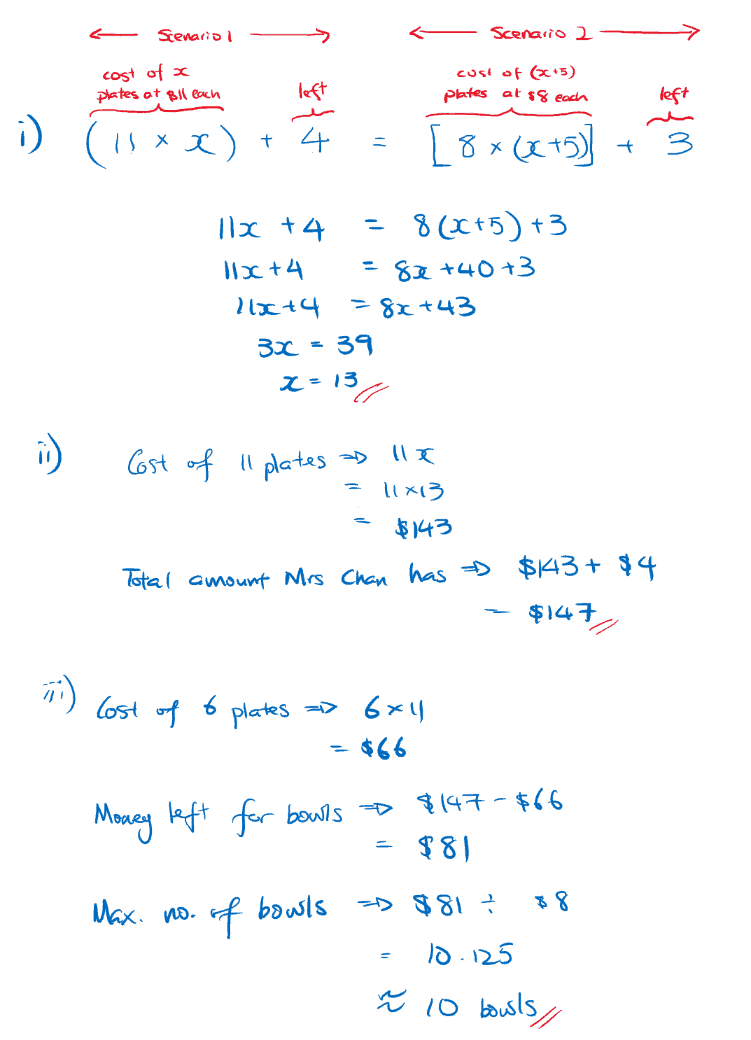# Question

This question is essentially a question on linear equations. We have the following equation

Mrs Chan’s original money = \$11 * x + \$ 4 = \$8 * (x + 5) + \$3

Using the laws of arithmetic (distributivity of multiplication over addition), this gives us:

Mrs Chan’s original money = \$(11x + 4) = \$ (8x + 8*5 +3) = \$(8x + 43)

We want to solve for x. Hence, we have apply laws of arithmetic:

11x + 4 = 8x + 43 => (11x + 4) – 8x = (8x + 43) – 8x =>

(3x + 4) = 43 => (3x +4) – 4 = 43 – 4 => 3x = 39 => (3x)/3 = 39/3 =>

x = 13.

Original Money = \$(11x + 4) = \$ (11*13+4) = \$ (143+4) = \$147.

let y be the max. amount of bowls she can buy. Then, (\$147 – \$6x) // \$(x+5) = y

Substituting x = 13, we have 69//18 = y.

y = 3 (because 4 * 18 = 72 > 69).